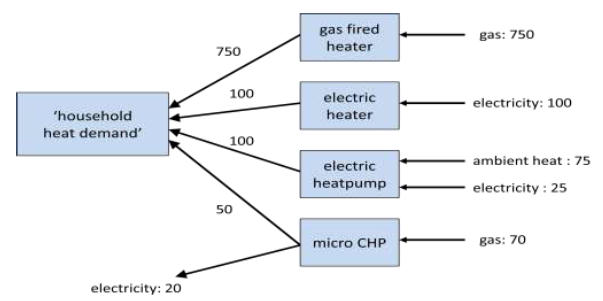# Useful demand

A good way to explain useful demand is the example of 'useful heat demand'.

In order to calculate how much would be saved with alternative heating technologies a useful heat demand is specified. This usable demand can usually not be found in literature or reports, so it is initially calculated based on the shares of the technologies used and their efficiencies. With this value (and the shares and efficiencies of the converters that supply this demand), the kernel can calculate how much of the energy carriers, such as gas and electricity, are used in total.

This should then match the final demand as supplied by the CBS. The value for usable heat demand cannot be verified directly, but when the values calculated for final demand match the verified CBS data the demand for ‘usable heat’ in reality is not really relevant.

An example is given in the figure below, where the useful ‘household heat demand’ can be calculated from the final demand. In this hypothetical example CBS states the final use for heating is 820 MJ gas and 125 MJ electricity. Assume that 75% of heat in households is supplied by gas fired heaters, 10% by electric heaters, another 10% by heat pumps and 5% by micro-CHPs. For sake of simplicity of this example we state the following: heaters are 100% efficient, the heat pump has a ‘Coefficient of Performance’ (CoP) of 4 and the micro-CHP heat/electricity ratio is 5 to 2.

Based on the shares and efficiencies, we can calculate that the heating demand is 1000 MJ and that 75 MJ ambient heat is ‘used’. The calculated usable demand now makes it easy to determine what the effect would be if shares or efficiencies of the heating technologies would change in the future.